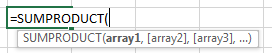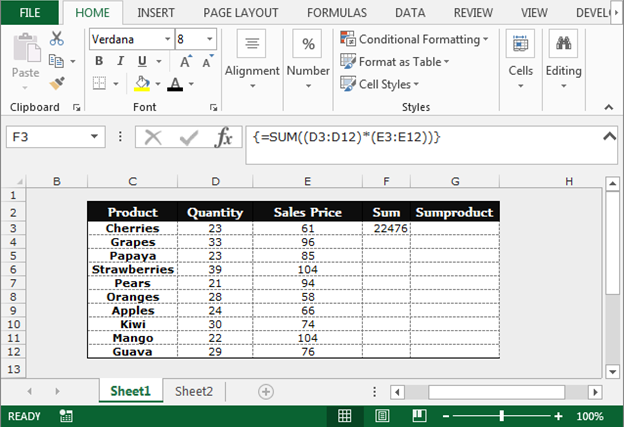# Simplifying complicated multiplication calculations using the SUMPRODUCT function

In this article, you will learn how to use Sumproduct function to simplifying the complicated multiplication calculations in Microsoft Excel.

What is Sumproduct function?

Sumproduct function is used to return the sum of products of corresponding ranges or array.First we will understand how and where we can use the SUMPRODUCT in place of another function.

Let’s take an example to understand:

We have a product list data in range C2:E12, Column C contains products, column D contains quantity, column E contains sales price. In order to return the total value of the product, we have used the SUM function.In cell G3 we will use Sumproduct function:-

Let’s understand how we can simplify complicated multiplication calculations using the SUMPRODUCT function.

• Select the cell G3.
• Write the formula.
• =SUMPRODUCT(D3:D12,E3:E12)
• Press Enter on your keyboard.
• The function will return the total value of the products.You can see that the results of both the formulas are same. In this way, SUMPRODUCT can simplify complicated multiplication calculations in Microsoft Excel.